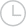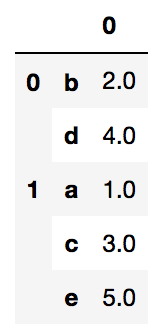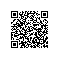# Pandas将groupby堆叠到DataFrame MultiIndex而不进行聚合-问答-阿里云开发者社区-阿里云

## Pandas将groupby堆叠到DataFrame MultiIndex而不进行聚合一码平川MACHEL2019-01-23 13:17:192659

from pandas import DataFrame as DF

df = DF.from_dict({'a':1, 'b':2, 'c':3, 'd':4, 'e':5}, orient='index')

df.groupby(lambda x: df0%2)

DF.from_dict({0:{'b':2,'d':4},1:{'a':1,'c':3,'e':5}},orient='index').stack().to_frame()（除了这一点，为什么值转换为浮点数？）

Python取消 提交回答

• 一码平川MACHEL
2019-07-17 23:26:34

使用pd.concat，它接受字典：

pd.concat({k: v for k, v in df.groupby(lambda x: df.loc[x, 0] % 2)})

`` 0``

0 b 2
d 4
1 a 1
c 3
e 5
迭代每个组并构建您的字典。可以使用字典理解来构造字典。

一个稍微快一点的解决方案，不涉及可调用，可以使用，

pd.concat({k: v for k, v in df.groupby(df.iloc[:,0] % 2)})

`` 0``

0 b 2
d 4
1 a 1
c 3
e 5
如果需要，可以尝试一下这个功能，

def add_level(df, grouper):

``````return pd.concat({k: v for k, v in df.groupby(by=grouper)})
``````

r = add_level(df, df.iloc[:,0] % 3)
add_level(r, r.iloc[:, 0] % 2)

``   0``

0 1 d 4
2 b 2
1 0 c 3
1 a 1
2 e 5

0 0使用钉钉扫一扫加入圈子
+ 订阅